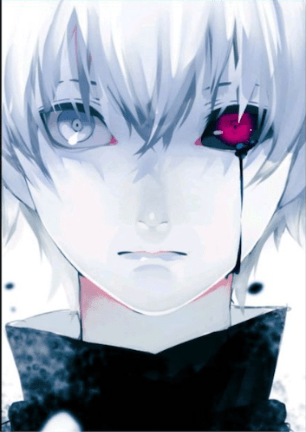# Implementing Filters in Phimp.me Android App

Image filters in phimp.me android app are implemented using the OpenCV library that enables the android developers to write code in C++/C which is compatible with java. I have implemented around eight filters in the filters.cpp file, mainly dealing with the colour variations that took place between the images.

Suppose that the color model used in consideration is the RGB (Red,Green,Blue) model and we define that every pixel is defined using these values. If we simply want to boost or focus on the red part of the image it can be done by enhancing the particular color in the source image i.e. modifying each pixel of the app by enhancing the blue color of each pixel. The code for the same can be written as :

```void applyBlueBoostEffect(cv::Mat &src, cv::Mat &dst, int val) {
register int x, y;
float opacity = val * 0.01f;
cvtColor(src, src, CV_BGRA2BGR);
dst = Mat::zeros(src.size(), src.type());
uchar r, g, b,val1;

for (y = 0; y < src.rows; y++) {
for (x = 0; x < src.cols; x++) {
r = src.at<Vec3b>(y, x);
g = src.at<Vec3b>(y, x);
b = src.at<Vec3b>(y, x);
val1 = saturate_cast<uchar>(b*(1+opacity));
if(val1 > 255) {
val1 = 255;
}
dst.at<Vec3b>(y, x) =
saturate_cast<uchar>(r);
dst.at<Vec3b>(y, x) =
saturate_cast<uchar>(g);
dst.at<Vec3b>(y, x) =
saturate_cast<uchar>(val1);
}
}
}
```

In the above function what we are doing is taking the source image and converting it to a matrix of known number of rows and columns. Now, we loop over this matrix created and at each

position in the matrix calculate the red, green and blue values present there. There is a parameterized value “val” that determines the amount to which the color chosen is enhanced.

A simple variable val1 contains the enhanced value of the color chosen, here which is color blue. We modify and boost the color by the equation  :

B = B*(1 + (0.01f* val) )

Finally at this particular position in the matrix all the three values of the color are updated i.e. the original red and green color but the modified blue color.

The output of this filter converts images as below shown :

Before                                                                                   AfterIn the above method defined we instead of enhancing one color we can even modify two colors or all the three at the same time to get different effects as per the required needs. An example where I implemented a filter to enhance two colors at the same time is given below, the purpose of this filter is to add a violet color tone in the image.

The code for the filter is :

```void applyRedBlueEffect(cv::Mat &src, cv::Mat &dst, int val) {
register int x, y;
float opacity = val * 0.01f;
cvtColor(src, src, CV_BGRA2BGR);
dst = Mat::zeros(src.size(), src.type());
uchar r, g, b;
uchar val1,val3;

for (y = 0; y < src.rows; y++) {
for (x = 0; x < src.cols; x++) {
r = src.at<Vec3b>(y, x);
g = src.at<Vec3b>(y, x);
b = src.at<Vec3b>(y, x);
val1 = saturate_cast<uchar>(r*(1+opacity));
if(val1 > 255) {
val1 = 255;
}

val3 = saturate_cast<uchar>(b*(1+opacity));
if(val3 > 255) {
val3 = 255;
}
dst.at<Vec3b>(y, x) =
saturate_cast<uchar>(val1);
dst.at<Vec3b>(y, x) =
saturate_cast<uchar>(g);
dst.at<Vec3b>(y, x) =
saturate_cast<uchar>(val3);
}
}
}
```

Here we can easily see that there are two values that are updated at the same time i.e. the red and the blue part. The resultant image in that case will have two color values updated and enhanced.

Similarly, instead of two we can also update all the three colors to get uniform enhancement across each color and the resultant image to have all the three colors with a boost effect overall.

The output that we get after the filter that boosts the red and blue colors of an image is  :

BEFOREAFTER